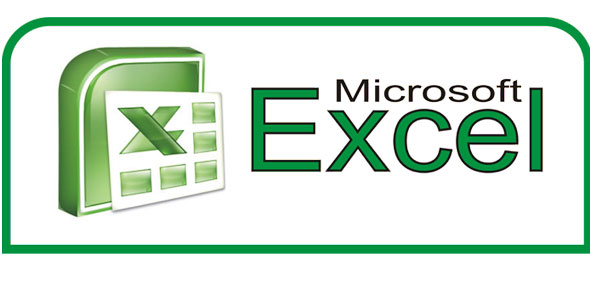20 Questions | Total Attempts: 1288SettingsMicrosoft Excel is a spreadsheet software which was developed by Microsoft. To become an expert one needs to master the basic formulas and functions used in the excel spreadsheet. MS Excel is mostly used for data processing, financial modelling and presentation. Take this quiz to test your knowledge and learn new formulas and functions used in excel. So, let's try out the quiz. Good Luck!

Related Topics
• 1.
• A.

=sumif

• B.

=sum

• C.

=

• D.

=sumif(

• 2.
Common Functions that we use most often are
• A.

=Sum

• B.

=Average

• C.

=Min

• D.

=Max

• E.

All of the above

• 3.
Excel aloows you to create ____________________________ that refer to column and row labels in place of the cell reference or range.
• A.

• B.

Natural Language Formulas

• C.

Arguments

• D.

Functions

• 4.
A block of cells in an Excel worksheet
• A.

Area

• B.

Range

• C.

Cell

• D.

Active cell

• 5.
A range of cells can be __________________or ____________________.
• A.

Connected, nonconnected

• B.

• C.

Contiguous, noncontiguous

• 6.
Worksheets that are selected as a unit; any action performed on this uit will affect all the worksheets in the group.
• A.

Active

• B.

Grouping

• C.

Attached

• 7.
A Range name may use up to ________ characters.
• A.

355

• B.

155

• C.

255

• D.

555

• 8.
The format for an IF statement is:
• A.

=SUM(condition,x,y)

• B.

=IF(condition,x,y)

• C.

=(condition,x,y)

• 9.
Correct format for a SUMIF statement is
• A.

=SUMIF(range, critera,sum_range)

• B.

=SUMIF(range,sum_range,critera)

• C.

=SUMIF(sum_range,critera,range)

• 10.
A graphic that allows you to compare and contrast data in a visual format.
• A.

Plot

• B.

Legend

• C.

Chart

• 11.
All charts use axes except
• A.

Bar

• B.

Line

• C.

Plot

• D.

Pie

• 12.
A chart actually has two backfrounds: the larger _______ _______ and the smaller ______ ______ which can be formmatted.
• A.

Tick mark, background

• B.

Chart area, plot area

• C.

Data axis, value axis

• 13.
A chart that occupies its own worksheet
• A.

Embedded chart

• B.

Chart sheet

• C.

Stand alone chart

• 14.
A key that identifies each of the data series in a chart.
• A.

Key

• B.

Map

• C.

Legend

• 15.
Name the three parts that are typically found in a chart.
• A.

Data series, Series labels and Catefory labels

• B.

Name box, value labels, name labels

• C.

X axis, y axis, z axis

• 16.
A ______________ends the argument.
• A.

Quotation mark

• B.

Closed parenthesis

• C.

Comma

• D.

Period

• 17.
Name three rules for naming ranges
• A.

• B.

Spaces are not allowed, do not start with range names, may include letters, numbers, underscores,blackslashes

• C.

• 18.
To display a comment in a worksheet
• A.

Roll mouse over red triangle

• B.

Open comment cell

• C.

Must highlight cell

• 19.
What key should be used to change the content of a cell?
• A.

F1

• B.

F2

• C.

F3

• D.

F4

• 20.
What function offers you the possibility to view different results depending on the entered condition?
• A.

=If()

• B.

=Sum()

• C.

=SumIf()

• D.

=TrueFalse()